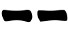# Paper Die (1d4)

In the first post about paper dice, I said I was not satisfied with the version that was marked to generate hexagrams with yarrow stalks probabilities: it could lead to getting too many (or too few) moving yin lines.
I found that the solution to this problem is to consider the paper die as having only four possible outcome (instead of eight) and use the same mechanic of the one card method.
I created another sheet to be printed for your convenience but you can simply fold the die from a blank sheet and draw the dots with a pen.
Each strip (remember: to be split in two squares) would appear like this:

After folding the die, two faces will have 4 dots, one face will have three dots and one will have five dots of which one red. If you are going to draw the dots with a pen, leave the central dot white and fill the other ones (or do the opposite).

To cast a line you need two operations. Proceed as follows:
1. Without looking, turn and roll the die in your hand;
2. When you feel the time is right, pick one of the faces and write down the number of black dots (either 3 or 4) you see;
3. Repeat step 1;
4. When you feel the time is right, pick one of the faces and write down the number of dots, regardless the color, (either 3, 4 or 5) you see;
5. Sum up the numbers and draw the line according the following table:
 6 7 8 96. Repeat steps 1-5 other five times and draw theline from th bottom to the top of the hexagram.
Probabilities
The faces are marked so that the first time we can get four possible outcomes: 3,4,4,4 while the second time we can get 3,4,4,5. Summing up as shown in the following table:

gives the same probabilities of the yarrow stalks method:
Prob(6) = 1/16 = 6.25%
Prob(8) = 7/16 = 43.75%
Prob(7) = 5/16 = 31.25%
Prob(9) = 3/16 = 18.75%
Prob(yin) = Prob(yang) = 1/2

#### Variants

Should you like the method but wanting the three coins pronbabilities you can use exactly the same method but marking the faces as follows:

Note that now there are two red dots in the group of five.
A file ready to be print is available (remember to print it at 100% of its size, with no automatic resize).